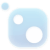# Top 20 NuGet mpfr Packages

The MPFR Native Interface for .NET Library exposes to .NET (through P-Invoke and .NET types) all of the functionality of the GNU MPFR Library (version 4.0.0). It automatically loads at runtime the 32-bit or 64-bit GNU MPFR library that matches the current CPU architecture, thus allowing building Vis...## The C++ Mathematical Expression Toolkit Library (ExprTk)

The C++ Mathematical Expression Toolkit Library (ExprTk) is a simple to use, easy to integrate and extremely efficient run-time mathematical expression parsing and evaluation engine. The parsing engine supports numerous forms of functional and logic processing semantics and is easily extendible. ht...
The C++ Mathematical Expression Toolkit Library (ExprTk) Extra Examples https://www.partow.net/programming/exprtk/index.html
A .NET wrapper for the GNU MPFR library.